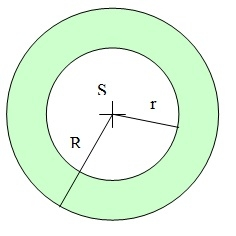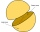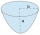# Concentric circles

There is given a circle K with a radius r = 8 cm. How large must a radius have a smaller concentric circle that divides the circle K into two parts with the same area?

Correct result:

r2 =  5.657 cm

#### Solution:

$r=8 \ \text{cm} \ \\ \ \\ S=\pi \cdot \ r^2=3.1416 \cdot \ 8^2 \doteq 201.0619 \ \text{cm}^2 \ \\ \ \\ S_{2}=S/2=201.0619/2 \doteq 100.531 \ \text{cm}^2 \ \\ \ \\ S_{2}=\pi \cdot \ r_{2}^2 \ \\ \ \\ r_{2}=\sqrt{ S_{2}/\pi }=\sqrt{ 100.531/3.1416 }=4 \ \sqrt{ 2 }=5.657 \ \text{cm}$Our examples were largely sent or created by pupils and students themselves. Therefore, we would be pleased if you could send us any errors you found, spelling mistakes, or rephasing the example. Thank you!

Leave us a comment of this math problem and its solution (i.e. if it is still somewhat unclear...):Be the first to comment!#### You need to know the following knowledge to solve this word math problem:

We encourage you to watch this tutorial video on this math problem:

## Next similar math problems:

• Folding tableThe folding kitchen table has a rectangular shape with an area of 168dm2 (side and is 14 dm long). If necessary, it can be enlarged by sliding two semi-circular plates (at sides b). How much percent will the table area increase? The result round to one-hu
• Pentagonal prismThe regular pentagonal prism is 10 cm high. The radius of the circle of the described base is 8 cm. Calculate the volume and surface area of the prism.
• FlowerbedWe enlarge the circular flower bed, so its radius increased by 3 m. The substrate consumption per enlarged flower bed was (at the same layer height as before magnification) nine times greater than before. Determine the original flowerbed radius.
• The basesThe bases of the isosceles trapezoid ABCD have lengths of 10 cm and 6 cm. Its arms form an angle α = 50˚ with a longer base. Calculate the circumference and content of the ABCD trapezoid.
• Isosceles triangle 9Given an isosceles triangle ABC where AB= AC. The perimeter is 64cm and altitude is 24cm. Find the area of the isosceles triangle
• Rectangle fieldThe field has a shape of a rectangle having a length of 119 m and a width of 19 m. , How many meters have to shorten its length and increase its width to maintain its area and circumference increased by 24 m?
• A concrete pedestalA concrete pedestal has a shape of a right circular cone having a height of 2.5 feet. The diameter of the upper and lower bases are 3 feet and 5 feet, respectively. Determine the lateral surface area, total surface area, and the volume of the pedestal.
• Two hemispheresIn a wooden hemisphere with a radius r = 1, a hemispherical depression with a radius r/2 was created so that the bases of both hemispheres lie in the same plane. What is the surface of the created body (including the surface of the depression)?
• A mapA map with a scale of 1: 5,000 shows a rectangular field with an area of 18 ha. The length of the field is three times its width. The area of the field on the map is 72 cm square. What is the actual length and width of the field?
• Rectangular base pyramidCalculate an area of the shell of the pyramid with a rectangular base of 2.8 m and 1.4 m and height 2.5 meters.
• Two bodiesThe rectangle with dimensions 8 cm and 4 cm is rotated 360º first around the longer side to form the first body. Then, we similarly rotate the rectangle around the shorter side b to form a second body. Determine the ratio of surfaces of the first and seco
• The tractorThe tractor sows an average of 1.5 ha per hour. In how many hours does it sows a rectangular trapezoid field with the bases of 635m and 554m and a longer arm 207m?
• Wooden containerThe cube-shaped wooden container should be covered with a metal sheet inside. The outer edge of the container is 54cm. The wall thickness is 25 mm. The container has no lid. Calculate. How many sheets will be needed to cover it?
• The tentCalculate how much cover (without a floor) is used to make a tent that has the shape of a regular square pyramid. The edge of the base is 3 m long and the height of the tent is 2 m.
• Base of prismThe base of the perpendicular prism is a rectangular triangle whose legs length are at a 3: 4 ratio. The height of the prism is 2cm smaller than the larger base leg. Determine the volume of the prism if its surface is 468 cm2.
• Squares above sidesTwo squares are constructed on two sides of the ABC triangle. The square area above the BC side is 25 cm2. The height vc to the side AB is 3 cm long. The heel P of height vc divides the AB side in a 2: 1 ratio. The AC side is longer than the BC side. Calc
• The parabolic segmentThe parabolic segment has a base a = 4 cm and a height v = 6 cm. Calculate the volume of the body that results from the rotation of this segment a) around its base b) around its axis.